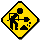I started this page on 22/2/05 so it doesn't have much on it.  I plan to more a lot more information here over the next couple of weeks.

# Prime Numbers

One of the most fascinating areas of mathamatics is that of prime numbers. Prime numbers are the building blocks of the positive integers and are comparable to the elements in chemistry.

Definition : A prime number is a positive integer not divisible without a remainder by any positive integer other than itself and one.

Although the definition of a prime number is simple enough, they present a series of questions which still proove to baffle even the most brilliant mathamatical brains. The most baffling and probally the most important questions concern both the distribution of the primes and the factoring of composite numbers into primes. Factoring is of particular importance as the worlds most secure computer systems rely on its difficulty.

A second page on primes, which shows a different more interesting way to draw them, can be found here.

## Drawing Prime Numbers Islands

Possibly the most pointless thing you can do with primes numbers is producing prime number islands. These are made by taking a list of prime numbers and then performing a mod 5 on each number in the list. Depending on the result of the mod, either 1, 2, 3, or 4 (except for the prime number 5, which returns 0), I move the drawing location either North, East, South or West (1 pixel at a time). I then draw a point at the new position. If the new position already has point drawn in it, I simply increment the colour of that point by 1.

The following images show some of the results. I used a sieve program to generate lists of prime numbers. The images below have been resized and converted to JPG's. If you want to see the original results just click on any of the images. This will download a zip file which will contain an unmodified BMP file. The original images look better than the resized images, but they are quite big. Should you have any problems with the zip files you can download either RAR or JPG files at the bottom of the page. I would appreciate an email should you experience any problems, then I can try and fix them.

I wrote the plot program myself. You can download it as an executable or source code from here. You can then experiment with various prime numbers.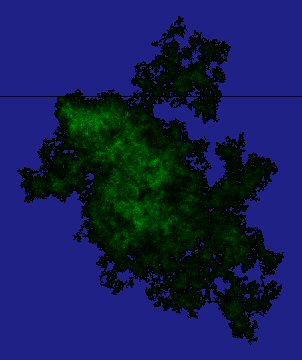All the prime numbers between 1 and 108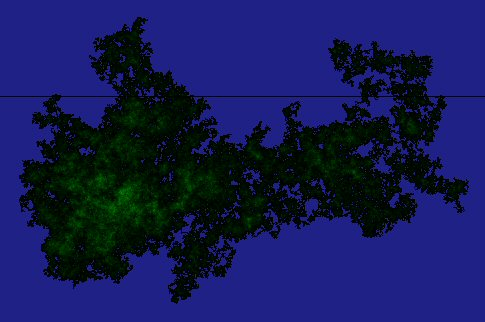All the prime numbers between 108 and 2*108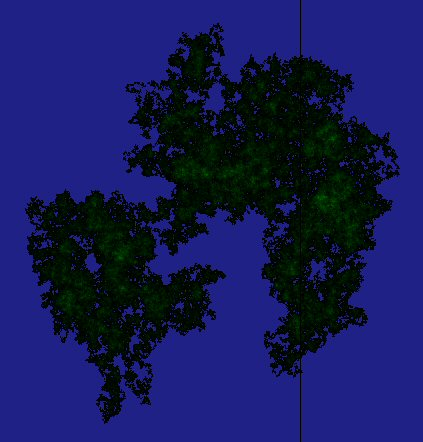All the prime numbers between 2*108 and 3*108All the prime numbers between 3*108 and 4*108All the prime numbers between 4*108 and 5*108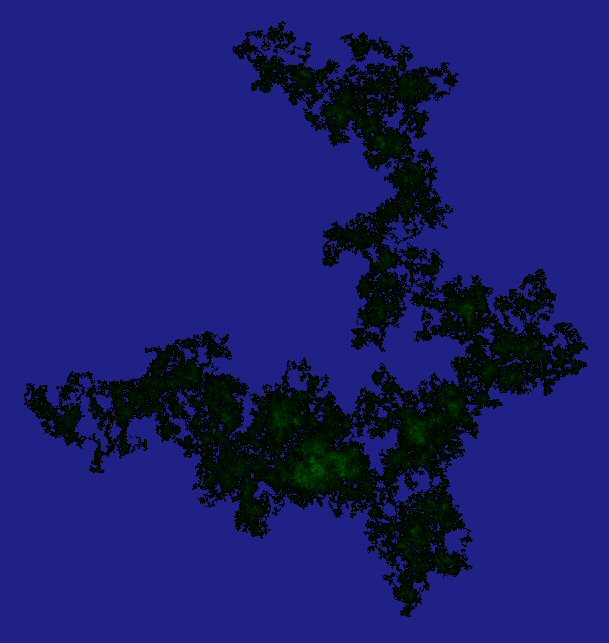All the prime numbers between 5*108 and 6*108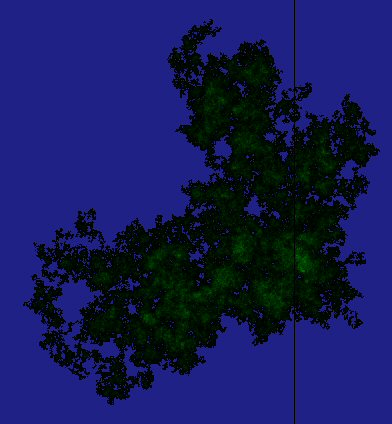All the prime numbers between 6*108 and 7*108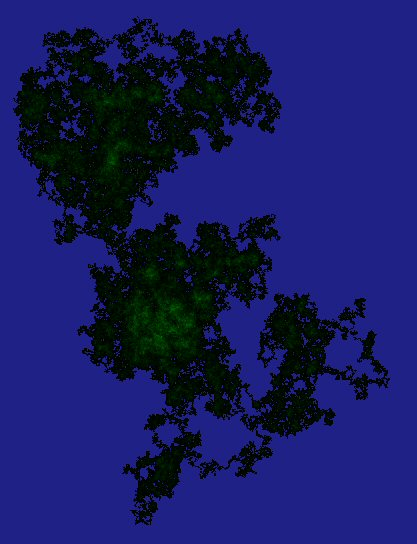All the prime numbers between 7*108 and 8*108